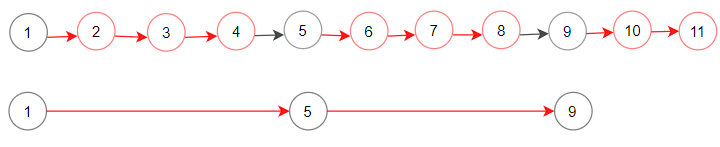Formatted question description: https://leetcode.ca/all/1474.html

# 1474. Delete N Nodes After M Nodes of a Linked List

Easy

## Description

Given the head of a linked list and two integers m and n. Traverse the linked list and remove some nodes in the following way:

• Keep the first m nodes starting with the current node.
• Remove the next n nodes
• Keep repeating steps 2 and 3 until you reach the end of the list.

Return the head of the modified list after removing the mentioned nodes.

Follow up question: How can you solve this problem by modifying the list in-place?

Example 1:Input: head = [1,2,3,4,5,6,7,8,9,10,11,12,13], m = 2, n = 3

Output: [1,2,6,7,11,12]

Explanation: Keep the first (m = 2) nodes starting from the head of the linked List (1 ->2) show in black nodes.

Delete the next (n = 3) nodes (3 -> 4 -> 5) show in read nodes.

Continue with the same procedure until reaching the tail of the Linked List. Head of linked list after removing nodes is returned.

Example 2:Input: head = [1,2,3,4,5,6,7,8,9,10,11], m = 1, n = 3

Output: [1,5,9]

Explanation: Head of linked list after removing nodes is returned.

Example 3:

Input: head = [1,2,3,4,5,6,7,8,9,10,11], m = 3, n = 1

Output: [1,2,3,5,6,7,9,10,11]

Example 4:

Input: head = [9,3,7,7,9,10,8,2], m = 1, n = 2

Output: [9,7,8]

Constraints:

• The given linked list will contain between 1 and 10^4 nodes.
• The value of each node in the linked list will be in the range [1, 10^6].
• 1 <= m,n <= 1000

## Solution

Create a dummy node that is before the head. Starting from the dummy node, each time move forward m steps, and then set the current node’s next node n steps ahead. Repeat the process until the end of the list is reached. Finally, return the head of the list.

/**
* Definition for singly-linked list.
* public class ListNode {
*     int val;
*     ListNode next;
*     ListNode() {}
*     ListNode(int val) { this.val = val; }
*     ListNode(int val, ListNode next) { this.val = val; this.next = next; }
* }
*/
class Solution {
public ListNode deleteNodes(ListNode head, int m, int n) {
ListNode dummyHead = new ListNode(0);
ListNode temp = dummyHead;
while (temp != null && temp.next != null) {
for (int i = 1; i <= m && temp != null && temp.next != null; i++)
temp = temp.next;
for (int i = 1; i <= n && temp != null && temp.next != null; i++)
temp.next = temp.next.next;
}